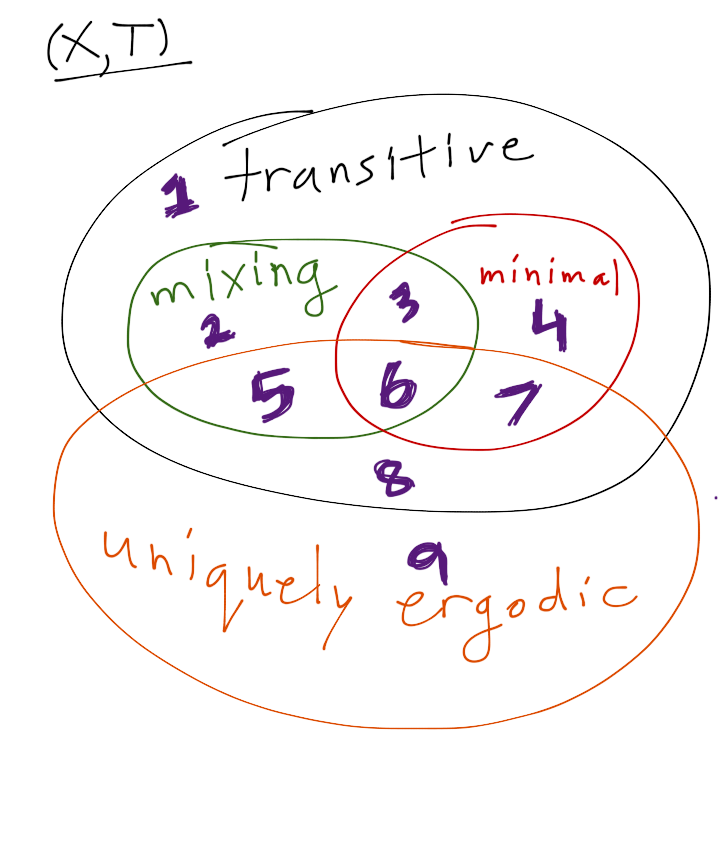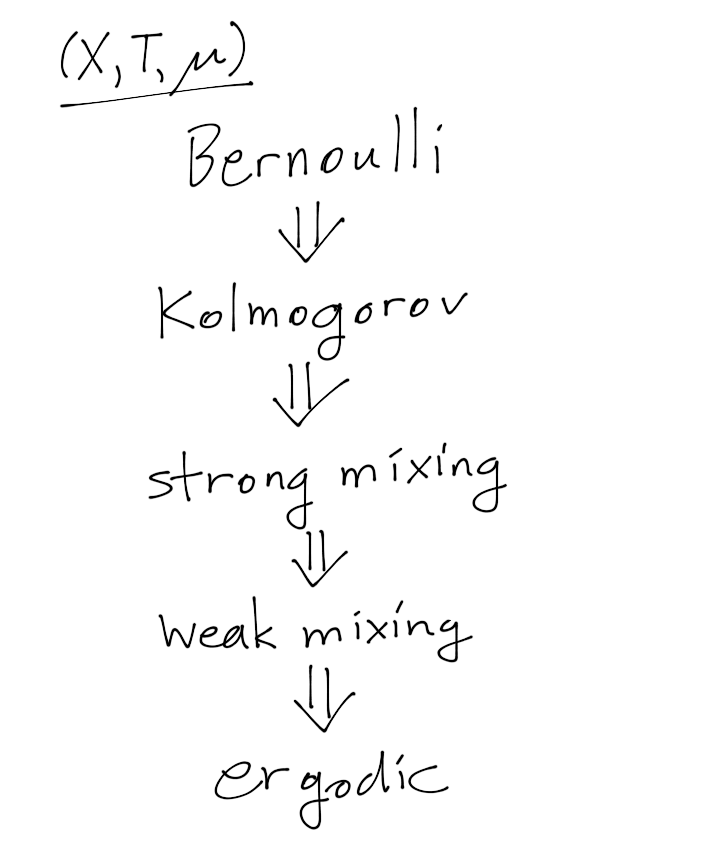Connection between properties of Dynamical and Ergodic Systems

Hi All

While studying Topological and Ergodic Dynamics, I've got quite preplexed by the different Properties a system might have (minimality, regionally recurring, transitivity, mixing, ergodic, uniquely ergodic etc.). All of which basically has the sense instability but not always equivalent. Do any of you has a graphic summary of those properties, and in what cases do they imply each other?

• I recommend reading Weiss and Glasner article - "ON THE INTERPLAY BETWEEN MEASURABLE AND TOPOLOGICAL DYNAMICS". – Asaf Nov 24 '12 at 16:26

Edit: I've updated this answer to reflect the helpful comments made by Andres Koropecki and Ian Morris.

As the other answers mentioned, the first crucial distinction you must make is that some properties refer to a topological dynamical system $(X,T)$, while others refer to a measure-preserving dynamical system $(X,T,\mu)$. Thus there are two different sets of definitions. Let me attempt a sketch at some of the relationships within each set.

First suppose you have a topological dynamical system $(X,T)$. Then four of the key properties are topological transitivity, topological mixing, minimality, and unique ergodicity. The first three are related by

• topologically mixing $\Rightarrow$ topologically transitive;
• minimal $\Rightarrow$ topologically transitive.

Unique ergodicity is independent of those three properties. The picture is the following.Counterexamples 1-9 illustrating the strict containments are as follows. (These may not be the simplest or the earliest counterexamples in each case, and I welcome corrections or improvements. This is based on some quick googling for things not already in my memory, plus the helpful additions offered by commenters.)

1. $X = \Sigma_2 \times \{a,b\}$, the direct product of a full two-shift with a period-two orbit, where the dynamics is $\sigma\times S$, with $\sigma$ the shift map and $S$ the map interchanging $a$ and $b$.

2. $X=\Sigma_2$.

3. Constructed by Bassam Fayad, Topologically mixing and minimal but not ergodic, analytic transformation on $\mathbb{T}^5$, 2000.

4. Constructed by Furstenberg, Strict ergodicity and transformation of the torus, 1961.

5. An irrational flow on the torus, slowed down near a single point: see the comment below by Andres Koropecki.

6. As Ian Morris points out in the comments, the identity map on a singleton set works here. A less trivial example was given by Karl Petersen, A topologically strongly mixing symbolic minimal set, 1970.

7. Rotation of the circle by an irrational angle.

8. Direct product of the example from 5 with a periodic orbit. (Again as suggested by Andres in the comments.)

9. North-south map: a map $T\colon [0,1]\to [0,1]$ with fixed points at $0,1$ and such that $T(x) < x$ for all $x\in (0,1)$. Identify the endpoints $0$ and $1$ so that this is a uniquely ergodic circle map.

A couple things are probably worth pointing out.

• Terminology is not always uniform. For example one of the papers I referenced (I think Petersen's) uses "ergodic" in place of "topologically transitive", to highlight the analogy with the measure-preserving case. So people may sometimes use different words for the same thing.
• Conversely the same word may mean different things. There are two definitions of topological transitivity, one involving open sets ($f^n(U) \cap V \neq \emptyset$ for some large $n$) and the other involving existence of a dense orbit. The definition involving open sets more closely mirrors the definition of topological mixing (non-empty intersection for every large $n$), while the definition with a dense orbit more closely mirrors minimality (denseness of every orbit). The definitions are equivalent if $X$ is separable, second category, and has no isolated points.

All of the above is for topological dynamical systems, where no invariant measure is specified. Then there are the ergodic properties: those that depend on a system preserving an measure $\mu$. For these one has the ergodic hierarchy.It is very often the case that one wishes to study a topological dynamical system as a measure-preserving system by equipping it with an invariant measure, and in this case it is quite reasonable to ask about the relationships between the two different classes of properties. But this depends on which invariant measure you choose, because in general there may be very many of them. One may ask what properties of $(X,T)$ let you pick invariant measures $\mu$ with certain nice properties, and this is a whole different story which would expand this answer far beyond the bounds of propriety.

• Wow - that's impressive! I would just add that there is ergodic theory beyond finite invariant measures - even for a single transformation. In particular, there are ergodic properties which don't make much sense for transformations with a finite invariant measure - like conservativity vs dissipativity dichotomy (the latter corresponds to proper discontinuity in the topological category). – R W Nov 24 '12 at 23:38
• For 5: take the vector field on $\mathbb{T}^2=\mathbb{R}^2/\mathbb{Z}^2$ given by $V(x) = \phi(x)v$ where $v$ is a vector of irrational slope and $\phi\colon \mathbb{T}^2\to \mathbb{R}$ is a map such that $\phi(x)>0$ for all $x\neq x_0$, $\phi(x_0)=0$. If $\phi$ is smooth (or at least if it goes to $0$ fast enough near $x_0$) you can show that the time-one map of $V$ is uniquely ergodic (the unique invariant measure being the Dirac measure at $x_0$). This type of map is topologically mixing as well, but not minimal. – Andres Koropecki Nov 25 '12 at 15:27
• For 8: You could do something similar to what you did in 1: Let $X=\mathbb{T}^2\times \{a,b\}$, where the dynamics on $X$ is given by the map from my previous example on the first coordinate and a swap of $a$ and $b$ on the second coordinate (a period two orbit). But I consider this cheating: I would like to see an example on a connected manifold. – Andres Koropecki Nov 25 '12 at 15:32
• In 9: I assume you are identifying the two endpoints of the interval, right? So rather than a north-south map this is a south-south map on the circle (with a saddle node). – Andres Koropecki Nov 25 '12 at 15:34
• 8: A set with two points in it, and a transformation which maps both points to the same point. If you want a homeomorphism, use a map with one point and one homoclinic orbit. – Ian Morris Nov 29 '12 at 16:11

Logically these are two different subjects.

1. Ergodic theory is about transformations of a space equipped with a measure (and the transformation preserves this measure). The measure is given in advance. The last 3 notions you mention are related to this situation, and express the various degrees of "mixing".

2. Topological dynamics is about continuous transformations of a topological space. The first 3 notions you mention are related to "mixing" in the topological sense. In general, there is no measure given in advance.

In many interesting situations BOTH structures are present: topology and measure. Actually both theories have their origin in Classical mechanics, where we have a smooth transformation of a manifold, which also preserves a measure on this manifold.

In general, if you have a continuous transformation of a topological space, no measure is given. And vice versa, when you have a transformation of a measure space, this space may not be equipped with any topology. (Like in probability).

In topological dynamics, when the measure is not given a priori, but the transformation is chaotic in the topological sense, it is possible to INTRODUCE an invariant measure, related to your transformation, and to bring the methods of ergodic theory to the study of smooth dynamics. Invariant measures exist for continuous transformations of a compact space, but there are very many of them. (This is called the Bogoliubov-Krylov theorem).

One famous way to introduce a "nice" invariant measure, intrinsically related to a smooth transformation is called the SRB-measure (Sinai-Ruelle-Bowen)

On all this, I recommend Sinai's books. They explain the ergodic theory itself, but also its applications to smooth dynamics.

• Of course your answer is very much in place. Though, in a lot of important examples (metric measurable spaces, localy compact groups) both structure exsits and therefore there's a point in regarding the relationship betwin both. In anyway, my question was about a graphic "mapping" of the different properties and their relations to each other. – amir sagiv Nov 24 '12 at 17:05
• "Both structures exist" is not enough. Your transformation should preserve both. Typically, you start with a smooth transformation, and the manifold has a measure or a class of natural measures, and you ask whether there exists a measure of this class which is invariant under your transformation. But "pure ergodic theory" can be developed without any topology, and "pure topological dynamics" without any measure. – Alexandre Eremenko Nov 24 '12 at 18:48

Generally, ergodic and topological properties are not related, apart from obvious implications. To give you an extreme example, there are actions on compact sets which are minimal, but in spite of that they are completely dissipative (i.e., are as far from ergodicity as possible) with respect to non-atomic quasi-invariant measures. The reason is that, roughly speaking, topological properties describe what happens with open and closed sets, without saying anything about general measurable sets.

Nonetheless, there are systems, for which topological and ergodic properties are related much closer. The classical example is provided by the geometric flows (geodesic and horocycle ones) on surfaces of constant negative curvature.